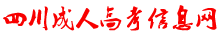﻿ 【河南专升本】数学1--一元函数积分学知识点睛（定积分的应用）-河南成人高考信息网# 【河南专升本】数学1--一元函数积分学知识点睛（定积分的应用）x=ax=b

2）作穿过平面图形且平行于轴的有向直线，进入区域交的是

y=f(x)，出来区域交的是y=f(x)y=dy=c

2）作穿过平面图形且平行于轴的有向直线，进入区域交的是

x=j(y)，出来区域交的是x=j(y)y=f(x)y=f(x)x=ax=b.

(2)确定在x轴上的投影区间：(3)确定上下曲线(4)计算积分：(1)画图.

(2)确定在x轴上的投影区间:[0,1].

(3)确定上下曲线:.

(4)计算积分x=j(y)x=j(y)y=dy=c.

(2)确定在y轴上的投影区间：(3)确定左右曲线x=j(y)x=j(y)

(4)计算积分：(1)画图

(2)确定在y轴上的投影区间：[-2,4]

(3)确定左右曲线(4)计算积分：### 河南成考交流群### 扫一扫加入考生微信群

#### 最新信息榜